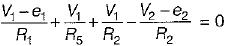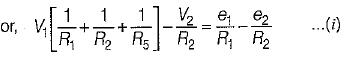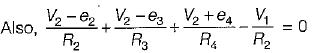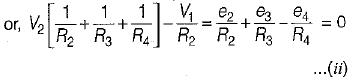Courses

# Basic Nodal & Mesh Analysis - 2

## 10 Questions MCQ Test Topicwise Question Bank for Electrical Engineering | Basic Nodal & Mesh Analysis - 2

Description
This mock test of Basic Nodal & Mesh Analysis - 2 for Electrical Engineering (EE) helps you for every Electrical Engineering (EE) entrance exam. This contains 10 Multiple Choice Questions for Electrical Engineering (EE) Basic Nodal & Mesh Analysis - 2 (mcq) to study with solutions a complete question bank. The solved questions answers in this Basic Nodal & Mesh Analysis - 2 quiz give you a good mix of easy questions and tough questions. Electrical Engineering (EE) students definitely take this Basic Nodal & Mesh Analysis - 2 exercise for a better result in the exam. You can find other Basic Nodal & Mesh Analysis - 2 extra questions, long questions & short questions for Electrical Engineering (EE) on EduRev as well by searching above.
QUESTION: 1

### For the circuit shown below, the value of current through RL is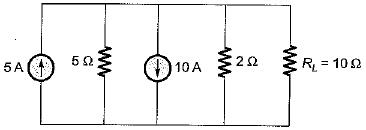Solution:

The given circuit can be reduced by combining resistors and current sources as shown below.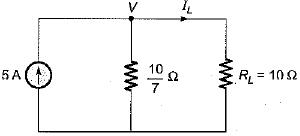Let the node voltage be V volts.
Applying nodal analysis, we have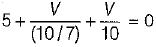or,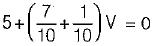or,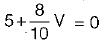or, 5 +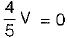or,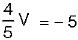or,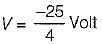So,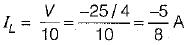QUESTION: 2

Solution:
QUESTION: 3

### In the node voltage technique of solving networks, choice of a reference node does not 1. affect the operation of the circuit.​ 2. change the voltage across any element. 3. affect the pd between any pair of nodes. 4. affect the voltages of various nodes. Q. Which of the above statements is/are true?

Solution:
QUESTION: 4

Nodal analysis is based on

Solution:
QUESTION: 5

RA and RB are the input resistances of circuits as shown below. The circuits extend infinitely in the direction shown. Which one of the following statements is TRUE?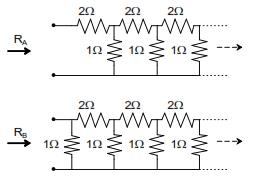Solution:
QUESTION: 6

In the circuit shown below, the value of lx is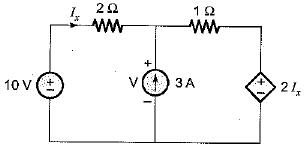Solution:

Applying nodal analysis at the given node, we have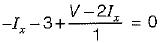or, 3Ix = V - 3 ...(i)
Also, 10 = 2Ix + V
or, V = 10 - 2Ix ...(ii)
Putting V from equation (ii) into equation (i),
3I= 10 - 2Ix - 3
or, 5Ix = 7
or,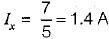QUESTION: 7

The value of V1 in the circuit shown in the given figure is​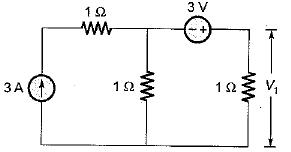Solution:

Let the node voltage be V' volts.
Applying nodal analysis at the given node, we have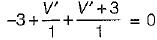or, 2V' = 0
or, V' = 0 volts
Now, V' = - 3 + 11
or, 0 = - 3 + V1
or, V1 = 3 volts

QUESTION: 8

Consider the electrical network shown below.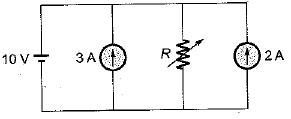What is the value of R so that current through the resistor R is zero?

Solution:

The given circuit can be redrawn as shown below (By combining current sources).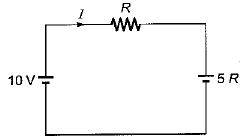Now, I = 0 (Given)
i.e.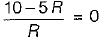or, R = 2Ω

QUESTION: 9

Assertion (A): In the method of solution of the network using nodal analysis, the assumption is made that the node potential is always higher than the other voltages appearing in the equation.
Reason (R): Nodal analysis is used to find the branch currents.

Solution:

Node potential is always higher than the other voltages appearing in the equations otherwise a negative value for current would result.

QUESTION: 10

The nodal equations of the circuit shown below are respectively given by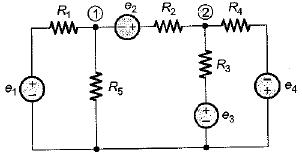Solution:

We have,